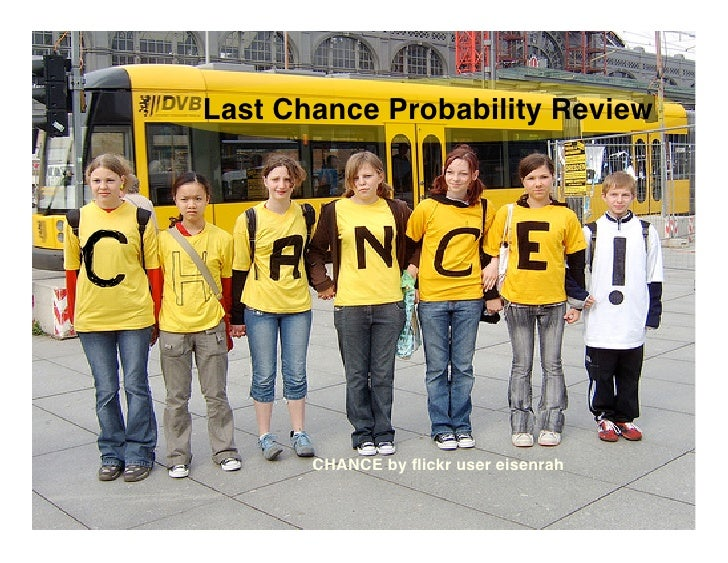Successfully reported this slideshow.Upcoming SlideShare
×

# Pre-Cal 40S June 2, 2009

1,766 views

Published on

More on medical testing, conditional probability, and probability and the binomial theorem.

Published in: Education, Health & Medicine
• Full Name
Comment goes here.

Are you sure you want to Yes No• Be the first to comment

• Be the first to like this

### Pre-Cal 40S June 2, 2009

1. 1. Last Chance Probability Review CHANCE by ﬂickr user eisenrah
2. 2. Suppose a test for cancer is known to be 98% accurate. This means that the outcome of the test is correct 98% of the time. Suppose that 0.5% of the population have cancer. What is the probability that a person who tests positive for cancer has cancer? Suppose 1 000 000 randomly selected people are tested. There are four possibilities: • A person with cancer tests positive • A person with cancer tests negative • A person without cancer tests positive • A person without cancer tests negative
3. 3. Suppose a test for cancer is known to be 98% accurate. This means that the outcome of the test is correct 98% of the time. Suppose that 0.5% of the population have cancer. What is the probability that a person who tests positive for cancer has cancer? (1) (a)How many of the people tested have cancer? (b) How many do not have cancer? (2) Assume the test is 98% accurate when the result is positive. (a) How many people with cancer will test positive? (b) How many people with cancer will test negative? (3) Assume the test is 98% accurate when the result is negative. (a) How many people without cancer will test positive? (b) How many people without cancer will test negative? (4) (a) How many people tested positive for cancer? (b) How many of these people have cancer? (c) What is the probability that a person who tests positive for cancer has cancer?
4. 4. Suppose a test for cancer is known to be 98% accurate. This means that the outcome of the test is correct 98% of the time. Suppose that 0.5% of the population have cancer. What is the probability that a person who tests positive for cancer has cancer? P(C|P) P(C|P)
5. 5. Suppose a test for cancer is known to be 98% accurate. This means that the outcome of the test is correct 98% of the time. Suppose that 0.5% of the population have cancer. What is the probability that a person who tests positive for cancer has cancer? P(H|N) P(H|N)
6. 6. Suppose a test for industrial disease is known to be 99% accurate. This means that the outcome of the test is correct 99% of the time. Suppose that 1% of the population has industrial disease. What is the probability that a person who tests positive has industrial disease?
7. 7. A couple intends to have four children. Assume that having a boy or a girl are equally likely events. Find the probability that the couple has at least two girls.
8. 8. A couple intends to have four children. Assume that having a boy or a girl are equally likely events. Find the probability that the couple has at least two girls.
9. 9. A couple intends to have four children. Assume that having a boy or a girl are equally likely events. Find the probability that the couple has at least two girls.